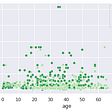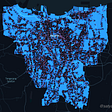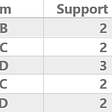# Statistical Sampling with Scikit-learn

Setting a test set is one of the early stages of developing a machine learning model. Thus creating a representative sample will determine how the model will perform in production.
The following example demonstrates the difference between stratified sampling and random sampling.

`import numpy as npimport pandas as pdfrom sklearn.model_selection import train_test_splitfrom sklearn.model_selection import StratifiedShuffleSplit`
1. First we create a population to work on:
`np.random.seed(42) # (1)p = 0.8a = ["Circle", "Square"]size = 100data = np.random.choice(a=a, size=size, p=[p, 1-p]) # (2)`
1. a seed, to make random numbers reproducible
2. the population of circles and squares, the sample size is 100 with a population of 0.8 and 0.2

2. Then, we convert the data to a data frame and process it:

`df = pd.DataFrame(data=data, columns=['X']) # (1)df.replace({'Circle': 0, 'Square': 1}, inplace=True) # (2)df['y'] = df.apply(lambda row: int(row["X"] == 0), axis=1) # (3)`
1. converting the data to a data frame
2. encoding the data

3. The random sampling step:

`X_train, X_test, y_train, y_test = train_test_split(df['X'], df['y'], test_size=0.2) # (1)p_random = X_test.value_counts() / len(X_test) # (2)`
1. sample size of 20%
2. p_random result is 0.85 and 0.15, a skewed sample

4. The stratified sampling step:

`split = StratifiedShuffleSplit(n_splits=1, test_size=0.2) # (1)for train_index, test_index in split.split(df, df['X']):    strat_train = df.loc[train_index]    strat_test = df.loc[test_index]p_strat = strat_test['X'].value_counts() / len(strat_test) # (2)`
1. sample size of 20%
2. p_strat result is 0.8 and 0.2, just like the original population.

In conclusion, the result is as expected. The stratified sampling was more representative than random sampling; this is only because the play was staged to demonstrates the differences. Increase the size of the population, and see what happens.

• The overall population is: 0.8, 0.2
• The random sample population is: 0.85, 0.15
• The stratified sample populations is: 0.8, 0.2

The stratified sampling function (StratifiedShuffleSplit) will return a sample from each stratum (in this case, our strata was column ‘X’).

Happy cross-validating!

The complete code is here https://github.com/booletic/medium/blob/main/strata.ipynb

--

--

--

## More from Mansoor Aldosari

https://github.com/booletic

Love podcasts or audiobooks? Learn on the go with our new app.

## Trap DS Projects: Beware of “Easy” Segmentation Projects## Search Autocomplete Personalisation## Visualization with Seaborn## Let’s Fix Jakarta’s Traffic## Google Cloud Professional Data Engineer Certification — My personal road map and thoughts in 2020## Frequent pattern mining, Association, and Correlations## Ratio Analysis — The Business Tool for Analyzing Financial Performance of A Business## Mansoor Aldosari

https://github.com/booletic

## What Could Go Wrong: Linear Regression## Logistic Regression- The history, the theory and the maths## Learning Ensemble methods## what is a regression in machine learning?